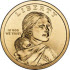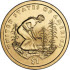# Random Variables

A Random Variable is a set of possible values from a random experiment.

### Example: Tossing a coin: we could get Heads or Tails.

Let's give them the values Heads=0 and Tails=1 and we have a Random Variable "X":In short:

X = {0, 1}

Note: We could choose Heads=100 and Tails=150 or other values if we want! It is our choice.

So:

• We have an experiment (such as tossing a coin)
• We give values to each event
• The set of values is a Random Variable

## Not Like an Algebra Variable

In Algebra a variable, like x, is an unknown value:

### Example: x + 2 = 6

In this case we can find that x=4

But a Random Variable is different ...

### Example: X = {0, 1, 2, 3}

X could be 0, 1, 2, or 3 randomly.

And they might each have a different probability.

## Capital Letters

We use a capital letter, like X or Y, to avoid confusion with the Algebra type of variable.

## Sample Space

A Random Variable's set of values is the Sample Space.### Example: Throw a die once

Random Variable X = "The score shown on the top face".

X could be 1, 2, 3, 4, 5 or 6

So the Sample Space is {1, 2, 3, 4, 5, 6}

## Probability

We can show the probability of any one value using this style:

P(X = value) = probability of that value

### Example (continued): Throw a die once

X = {1, 2, 3, 4, 5, 6}

In this case they are all equally likely, so the probability of any one is 1/6

• P(X = 1) = 1/6
• P(X = 2) = 1/6
• P(X = 3) = 1/6
• P(X = 4) = 1/6
• P(X = 5) = 1/6
• P(X = 6) = 1/6

Note that the sum of the probabilities = 1, as it should be.### Example: How many heads when we toss 3 coins?

X = "The number of Heads" is the Random Variable.

So the Sample Space = {0, 1, 2, 3}

But this time the outcomes are NOT all equally likely.

The three coins can land in eight possible ways:

 X =   "Number of Heads" HHH3 HHT2 HTH2 HTT1 THH2 THT1 TTH1 TTT0

Looking at the table we see just 1 case of Three Heads, but 3 cases of Two Heads, 3 cases of One Head, and 1 case of Zero Heads. So:

• P(X = 3) = 1/8
• P(X = 2) = 3/8
• P(X = 1) = 3/8
• P(X = 0) = 1/8### Example: Two dice are tossed.

The Random Variable is X = "The sum of the scores on the two dice".

Let's make a table of all possible values:

1st Die 1 2 3 4 5 2 3 4 5 6 7 3 4 5 6 7 8 4 5 6 7 8 9 5 6 7 8 9 10 6 7 8 9 10 11 7 8 9 10 11 12

There are 6 × 6 = 36 possible outcomes, and the Sample Space (which is the sum of the scores on the two dice) is {2, 3, 4, 5, 6, 7, 8, 9, 10, 11, 12}

Let's count how often each value occurs, and work out the probabilities:

• 2 occurs just once, so P(X = 2) = 1/36
• 3 occurs twice, so P(X = 3) = 2/36 = 1/18
• 4 occurs three times, so P(X = 4) = 3/36 = 1/12
• 5 occurs four times, so P(X = 5) = 4/36 = 1/9
• 6 occurs five times, so P(X = 6) = 5/36
• 7 occurs six times, so P(X = 7) = 6/36 = 1/6
• 8 occurs five times, so P(X = 8) = 5/36
• 9 occurs four times, so P(X = 9) = 4/36 = 1/9
• 10 occurs three times, so P(X = 10) = 3/36 = 1/12
• 11 occurs twice, so P(X = 11) = 2/36 = 1/18
• 12 occurs just once, so P(X = 12) = 1/36

## A Range of Values

We could also calculate the probability that a Random Variable takes on a range of values.

### Example (continued) What is the probability that the sum of the scores is 5, 6, 7 or 8?

In other words: What is P(5 ≤ X ≤ 8)?

P(5 ≤ X ≤ 8) =P(X=5) + P(X=6) + P(X=7) + P(X=8)
= (4+5+6+5)/36
= 20/36
= 5/9

## Solving

We can also solve a Random Variable equation.

### Example (continued) If P(X=x) = 1/12, what is the value of x?

Looking through the list above we find:

• P(X=4) = 1/12, and
• P(X=10) = 1/12

So there are two solutions: x = 4 or x = 10

Notice the different uses of X and x:

• X is the Random Variable "The sum of the scores on the two dice".
• x is a value that X can take.

## Continuous

Random Variables can be either Discrete or Continuous:

• Discrete Data can only take certain values (such as 1,2,3,4,5)
• Continuous Data can take any value within a range (such as a person's height)

All our examples have been Discrete.

## Mean, Variance, Standard Deviation

You can also learn how to find the Mean, Variance and Standard Deviation of Random Variables.

## Summary

• A Random Variable is a set of possible values from a random experiment.
• The set of possible values is called the Sample Space.
• A Random Variable is given a capital letter, such as X or Z.
• Random Variables can be discrete or continuous.